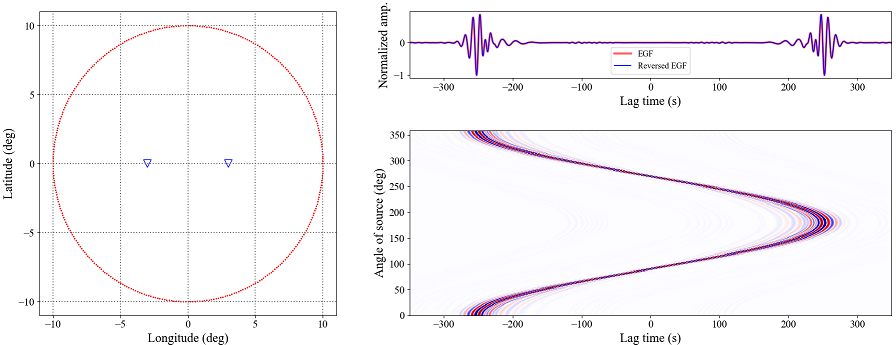New responses, if the wave field is diffuse, can be retrieved from interstaion cross-correlation of ambient noise. However, the cross-correlation time functions are asymmetric if the noise sources distribute unevenly. Here, we give you an example to show that how the cross-correlation functions vary with the angles of noise sources (red dots). We computed synthetic seismograms of earthquakes with angles from 1 to 360 degrees with an interval of 1 degree and data are recorded by two receivers (blue triangles); then we compute the cross-correlation time functions and align them with the angles; lastly, we stack all the cross-correlations to show you it’s symmetric under the condition that the noise sources distribute evenly.